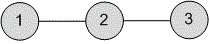bolt
Try our new interface for solving problems
Problems

# Is it a Tree?

Time limit 1 second
Memory limit 128 MiB

Undirected graph without loops and multiple edges is given with the adjacency matrix. Determine whether the graph is a tree.

## Input data

The first line contains the number of vertices n~(1 \le n \le 100) in the graph. In the next lines the adjacency matrix of size n \cdot n is given, where 1 denotes the presence of an edge, and 0 its absence. The matrix is symmetric with respect to the main diagonal.

## Output data

Print the message "YES", if the graph is a tree, and "NO" otherwise.## Examples

Input example #1
3
0 1 0
1 0 1
0 1 0

Output example #1
YES